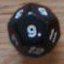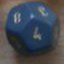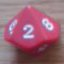Hints and tips

## Hints and tips

 Wed Jul 6 16:48:17 2011   by   Torben There is a limit iteration of 12 to accumulate loops and recursive functions, but not to repeat loops, as it is possible to calculate the probability distribution for repeat loops without unrolling the loop. So if you can, you should use repeat loops instead of recursion.The difference is most noticeable if many iterations are common, i.e, if the termination condition is likely to be false. Compare, for example, these two:`repeat x:=d10 until p={7,10}`which repeats until the result is either 7 or 10. As you would expect, both results have 50% probability.If you, instead, use functions:`function keepRolling(x)  = if x={7,10} then x else call keepRolling(d10)call keepRolling(d10)`You will find that both results now only have 46.564% chance of happening, which doesn't add up to 100%. The remaining 6.872% is the chance that the iteration/recursion limit is reached and an undefined result returned.If you get totals below 100%, this is an indication that the limit has been reached and that you should not treat the probabilities as exact. Fri Dec 27 07:52:54 2013   by   wyrdR I was looking for a way to pass a set of numbers to the offline version of Troll. In the online version, I would defined a set like this:`set:={1,7,2,9};`But if I needed to pass this via the command line it failed.Instead I came up with a function called split designed so you can send up to a set of 9 digits to provide a set of single digit numbers - e.g. `\$ troll 4 trollscript.t N=1 set=1729`Inside trollscript.t would be the function to split set into {1,7,2,9}.The split function:`function split(x)=   a:=x mod 10;   b:=x/10;  a U if (b>9) then call split(b) else bset:=call split(set);`To handle 2 digit numbers, you'd need to change the 10 to 100  instead, but this might limit the set to 4 or 5 digits. Sat Dec 28 01:19:54 2013   by   Torben That's rather clever, but it has a small flaw: If you give split a one-digit number, it will return the set containing 0 and that number.  For example, split(9) gives 0 9.To remedy this, you can modify the function to`function split(x)=  if x>9 then    {x mod 10, call split(x / 10)}  else x` Sun Dec 29 02:23:39 2013   by   wyrdR Thanks.Funny, I encountered this, but rather clumsily ran this on set afterwards:`set:=set drop {0};`Your solution is way more elegant! :)